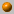##### Personal tools
You are here: Home Computational Geometry - Spring 2012
« February 2023 »
February
SuMoTuWeThFrSa
1234
567891011
12131415161718
19202122232425
262728

# Computational Geometry - Spring 2012

Instructor: Dan Halperin , danha AT post

Monday, 16:00--19:00
Kaplun 118

Office hours: Monday, 19:00--20:00

The course covers fundamental algorithms for solving geometric problems such as computing convex hulls, intersection of line segments, Voronoi diagrams, polygon triangulation, and linear programming in low dimensional space. We will also discuss several applications of geometric algorithms to solving problems in robotics, GIS (geographic information systems), computer graphics, and more.

### Bibliography:

The main textbook of the course is
Computational Geometry: Algorithms and Applications (CGAA), 2nd or 3rd edition
by M. de Berg, M. van Kreveld, M. Overmars, and O. Schwarzkopf.

A bibliographic list for the course

The standard assignments will account for 10% of the final grade, in case they improve the final grade. The grade in the final exam, to be held on Monday, July 2nd, 2012, will account for the remaning 90% of the grade in the course.

Although the assignment grades will be used only for improving the final grade, submission of the assignments is mandatory and a prerequisite for taking the exam.

new, June 24th, 2012: Exam(ple), pdf file

We encourage you to submit the programming assignment as well. This is not mandatory.
If however you do submit it, then your grade breakdown will be 10% the standard assignments,
10% the programming assignment, and 80% the final exam. Here as well, the assignments grades will
be counted toward the final grade only if they improve it.

Assignment no. 1 (pdf file), due: April 2nd, 2012.

Assignment no. 2 (pdf file), due: April 23rd, 2012.

Programming Assignment, revised 16/04/12, (pdf file), due: May 7th, 2012 (you may work and submit in pairs). Additional information.

Assignment no.3 (pdf file), due: May 14th, 2012

Assignment no.4 (pdf file), due: June 11th, 2012

### Course Summary:

Below you'll find a very brief summary of what was covered in class during the semester. This should not be taken as a complete description of the course's contents.

For an outline of the course, see for example, the course summary in the 2010 computational geometry course.5.3.12

What is Computational Geometry? Course outline, motivation and techniques. Naive convex hull algorithms (see Chapter 3 in O'Rourke's book). Orientation (Side-of-line) test. Gift wrapping. Graham's O(n log n) algorithm (Chapter 1 in CGAA)12.3.12

Graham's O(n log n) algorithm (repeat, Chapter 1 in CGAA). Lower bound for convex hull algorithms.

Output sensitive algorithm to compute the intersections of line segments: Bentley Ottmann's plane sweep. The doubly-connected edge list (DCEL). (Chapter 2 in CGAA).

Overlay of planar subdivisions (CGAA, Chapter 2), to be continued.19.3.12

Overlay of planar subdivisions, continued; Boolean operations on polygons (CGAA, Chapter 2).

A proof of Euler's formula (from "Proofs from The Book" by Aigner and Ziegler).
http://en.wikipedia.org/wiki/Ramer-Douglas-Peucker_algorithm.
whose References include the Hershberger-Snoeyink improvement that we disucssed).

Polygon triangulation; Art gallery problems - Part I
We have shown that \$\lfloor n/3 \rfloor\$ guards are sometimes necessary and always sufficient to guard a simple polygon with n vertices. We have shown that every simple polygon can be triangulated; that the triangulation graph is 3-colorable; that the dual of the triangulation graph is a tree.

The material covered in this lesson as well as in Part II next week, could be found in O'Rourke's book, Chapters 1 and 2, and in CGAA, Chapter 3.26.3.12

Polygon triangulation continued. Triangulating a monotone polygon in linear time.
Triangulating a simple polygon in O(n log n) time.

Talk by Karl Boehringer, University of Washington:
Nanomanufacturing by Bottom-up Growth: An Application of Voronoi Diagrams [slides]2.4.12

Polygon triangulation; Art gallery problems, continued.

We have shown that \Omega(n^{3/2} guards are sometimes needed to guard a polyhedron with n vertices. The construction is due to Seidel and its presentation is taken from the book Art Gallery Theorems and Algorithms by J. O'Rourke.

The casting problem. Half plane intersection. (CGAA, Chapter 4).

A brief introduction to CGAL (1 hour, Efi Fogel).4.4.12 (Wednesday)

A randomized algorithm for LP in 2D.  The minimum enclosing disk problem (CGAA, Chapter 4).16.4.12

Orthogonal Range Searching [slides].23.4.12

Orthogonal Range Searching, continued: query time bound for kd-trees (CGAA, Chapter 5).

Linear Programming, continued: unboundedLP2D; minimum enclosing disc  (CGAA, Chapter 4).30.4.12

Remarks on LP in higher dimensions.

Solution to Ex 3.4.

Introduction to point location and trapezoidal maps  (CGAA, Chapter 6).7.5.12

Trapezoidal maps and planar point location, continued. CGAA, Chapter 6.

Solution to Ex 3.1.

The video Objects that cannot be taken apart with two hands by Jack Snoeyink, from the 1993 Video Review in the 19th ACM Symposium on Computational Geometry.

Introduction to Voronoi diagrams.14.5.12

Voronoi diagrams of points in the plane: structure, complexity, anf Fortune's algorithm. CGAA, Chapter 7.

Bird's eye view: Voronoi diagrams of segments and polygons and their use for high-clearance motion planning; the medial axis of a polygon; the farthest-site Voronoi diagram; finding the minimum width annulus (CGAA, Chapter 7; O'Rourke's book, Chapter 5)21.5.12
Point-Line duality, arrangements of lines in the plane, the zone theorem and incremental construction of the arrangement. Application to computing the half-plane discrepancy in a square. CGAA, Chapter 8.4.6.12
Triangulations. Delaunay triangulations. CGAA, Chapter 9.11.6.12
The connections between: convex hulls and envelopes, Voronoi diagrams, Delaunay triangualtions.
##### Document Actions